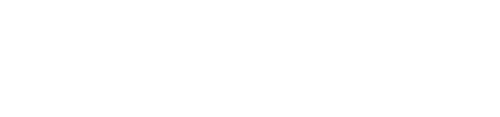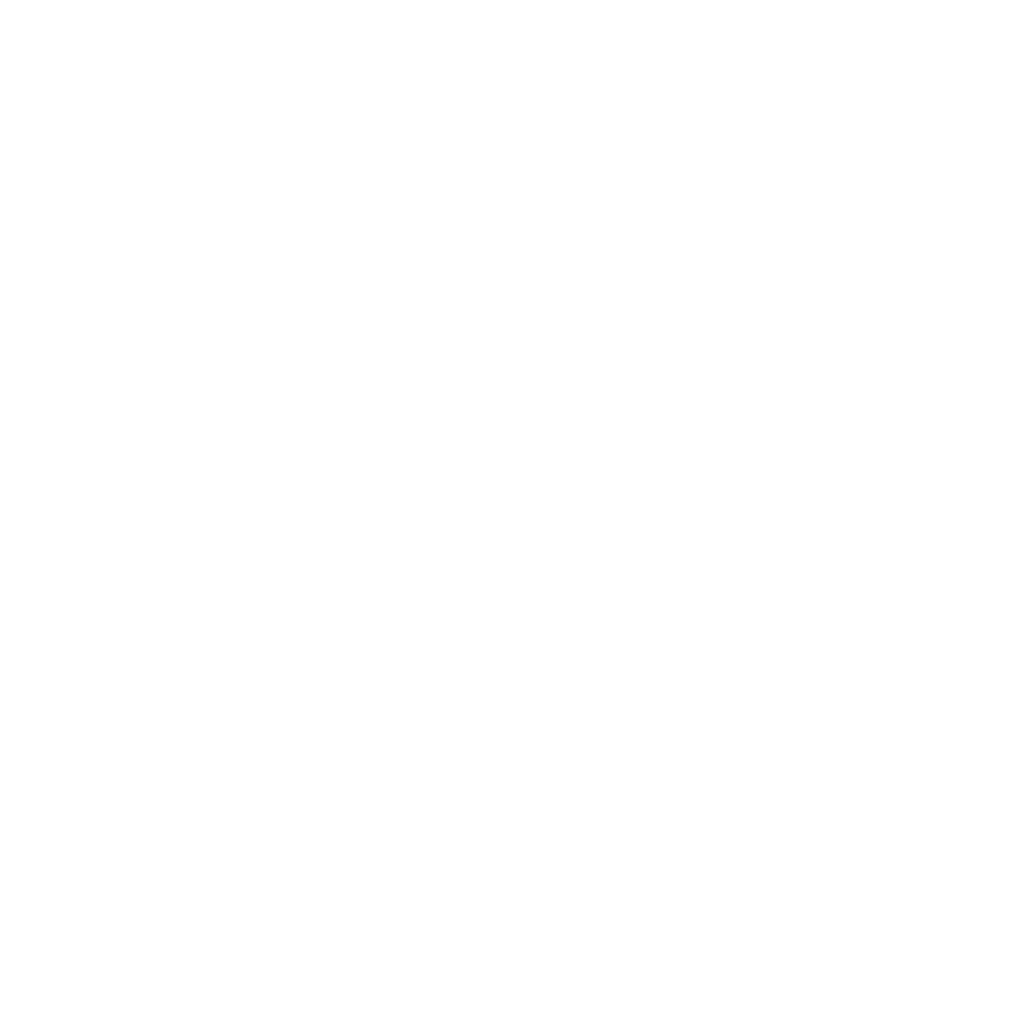## OVERVIEW

Geometry exists everywhere in the world around you. We use it to build bridges, to design maps, or to create perspective in paintings. Throughout this course, you will use problem solving and real-world applications to gain the knowledge of geometric concepts and their practical uses.

## MAJOR CONCEPTS

• Basics of Geometry
• Basic Constructions
• Constructing with Parallel and Perpendicular Lines
• Constructions with Technology
• Introduction to Proofs
• Translations
• Reflections
• Rotations
• Rigid Motion and Congruence
• Line and Angle Proofs
• Triangle Proofs
• Parallelogram Proofs
• Dilations
• Similar Polygons
• Similar Triangles
• Triangle Congruence and Similarity
• Applications of Congruence and Similarity
• Using the Coordinates
• Slope
• Coordinate Applications
• Solving Right Triangles
• Trigonometric Ratios
• Applying Trigonometric Ratios
• Formulas
• Applications of Volume
• Density
• 3-D Figures
• Properties of a Circle
• Inscribed and Circumscribed Circles
• Applications of Circles

## Fee Details

 Progam Regular Honors Fee Component Course Fee Amount (USD) \$ 558 \$ 583 Description To be paid by the student at the time of Enrollment.

## Requirements

Scientific Calculator

## Algebra 1 or its equivalent

Scroll to Top